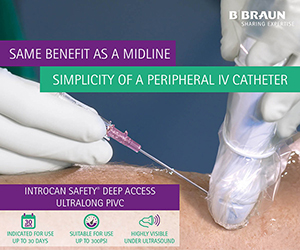# Equation for peripherally inserted central catheter length on the left side“The equation of our study would provide a new equation for proper estimation of catheter length in case of bedside insertion of left arm PICC in relation to height” Jeon et al (2014).

Reference:

Jeon, E-Y., Koh, S.H., Lee, I.J., Ha, H. and Park, B.J. (2014) Useful equation for proper estimate of left side peripherally inserted central venous catheter length in relation to the height. The Journal of Vascular access. October 16th. .

Abstract:

Purpose: Direct measurement of venous length is easy to cause contamination during bedside insertion of peripherally inserted central venous catheter (PICC). It is necessary to provide an equation for proper estimate of catheter length in case of bedside insertion of PICC in relation to patient height.

Methods: For 165 PICC cases through left arm vein in 151 adult patients (male: female = 72:79), the cubital crease to carina length (CCL) was calculated as follows: CCL = (distance from cubital crease to puncture point) + (length of PICC inside body) – (distance from carina to catheter tip on post-procedural chest radiograph). We analyzed the relationship between CCL and height with regression analysis and suggest a new equation of CCL based on height.

Results: The mean CCL through the left arm vein was 47.1 ± 2.6 cm in male and 44.0 ± 2.9 cm in female. CCL was significantly correlated with patient height. Equation of CCL (cm) based on height was as follows: CCL = height * 0.19 + 14.

Conclusions: The equation of our study would provide a new equation for proper estimation of catheter length in case of bedside insertion of left arm PICC in relation to height and be helpful for optimal positioning of catheter tip of PICC.

Thank you to our partners for supporting IVTEAM